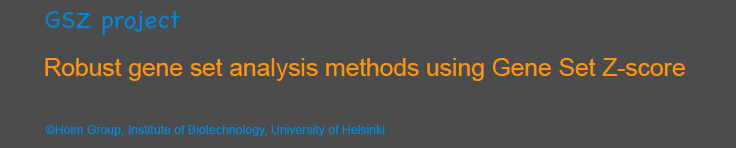# Gene set analysis of gene expression data with few replicates

(This is supplementary page for mGSZm.)

# Description:

Gene set analysis has become increasingly popular in bioscience research. Many gene set analysis methods have been developed since the introduction of the concept in the early 2000s. Most of the gene set analysis methods require that the gene expression data must have at least six biological replicates per experimental group. However, datasets with fewer biological replicates are prevalent, mostly for economical reasons. We have developed a gene set analysis method, mGSZm, that is applicable to multi-group gene expression dataset with as few as three replicates. Our method has shown the best performance as compared to the state-of-the-art gene set analysis methods with three different real gene expression datasets and evaluation methods.

mGSZm is based on Gene set Z-score, advanced permutation, and asymptotic P-value calculation.

Gene set Z-score: Gene set Z-score (GSZ) is a gene set scoring function based on solid statistics (Törönen et al.,2009). GSZ has been successfully used in various projects and is shown to produce very good results (PANNZER, Meta analysis of large set of gene expression data, Comparison of gene set methods, Analysis of Self-Organizing Maps (SOM) for enrichment signal).

Advanced permutation: Permutation methods are popular in gene set analysis for assigning P-value to gene set scores due to its ability to give reliable statistical inference without making strong distributional assumptions. Most of the gene set analysis methods are based on conventional permutation method that permutes only the sample groups that are being compared and ignores the other sample groups in the dataset. Thus, the conventional permutation method is not applicable to datasets with few biological replicates (less than six) as the number of unique permutations is too few to estimate P-values accurately. We have developed advanced permutation method for multi-group gene expression data with less than six replicates per group. We have shown that the advanced permutation method performs clearly better than the conventional permutation method.

Asymptotic P-value: Asymptotic P-value calculation involves fitting a suitable asymptotic model to the null distribution of gene set scores (Mishra et al.,2014). Asymptotic P-value calculation requires considerably fewer permutations as compared to the empirical P-value calculation to accurately estimate P-values and thus speeds up the gene set analysis process.

# R package implementing mGSZm:

Development of R package for mGSZm is ongoing. The preliminary R package for mGSZm can be downloaded from:

# Example run in R:

• ###############################################
• library(mGSZm)

# generate gene names

gene.names <- paste("g",1:100, sep = "")

# create random gene expression data matrix with three sample groups with three replicates per sample group

set.seed(100)

x <- matrix(rnorm(100*9),ncol=9)

rownames(x) <- gene.names

b <- matrix(2*rnorm(30),ncol=3)

ind <- sample(1:10,replace=FALSE)

x[ind,4:6] <- x[ind,4:6] + b

l <- c(rep(1,3),rep(2,3),rep(3,3))

# create random gene sets

y <- vector("list", 20)

for(i in 1:length(y)){

y[[i]] <- sample(gene.names, size = 10)

}

names(y) <- paste("set", as.character(1:20), sep="")

### Creating GSZ object of input data ###

gsz_obj <- GSZ(exprdat=x,genesets=y,sampleLabels=l)

### Running mGSZm function ###

res <- mGSZm(gsz_obj,comparisons=c("group1-group2","group1-group3"),perm.number=10)

### Returning data frame with top 'n' significant gene sets for selected or all comparisons ###

top_results <- topResults(res,comparison=c("group1-group2","group1-group3"), order.by="P-value",direction="mixed",n=10)

### For gene expression data with only two groups ###

### Modifying the sample labels of the gene expression data in order to make it two group gene expression data ###

sampleLabels(gsz_obj) <- c(1,1,1,1,1,2,2,2,2)

### Running mGSZm function ###

res_2grp <- mGSZm(gsz_obj,perm.number=10)

### Returning data frame with top 'n' significant gene sets ###

top_results <- topResults(res_2grp)